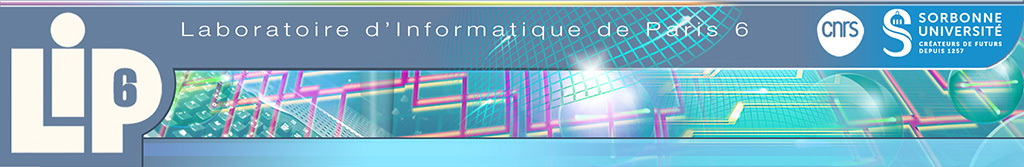Algorithms to Compute Chern-Schwartz-Macpherson and Segre Classes and the Euler Characteristic

06/27/2014
Speaker(s) : Martin Helmer (The Univ. of Western Ontario, Canada)
Let V be a closed subscheme of a n dimensional projective space. We give algorithms to compute the Chern-Schwartz-MacPherson class, Euler characteristic and Segre class of V. These algorithms can be implemented using either symbolic or numerical methods. The basis for these algorithms is a method for calculating the projective degrees of a rational map defined by a homogeneous ideal. When combined with formula for the Chern-Schwartz-MacPherson class of a projective hypersurface and the Segre class of a projective variety in terms of the projective degrees of certain rational maps this gives us algorithms to compute the Chern-Schwartz-MacPherson class and Segre class of a projective variety. Since the Euler characteristic of V is the degree of the zero dimensional component of the Chern-Schwartz-MacPherson class of V our algorithm also computes the Euler characteristic of V. The algorithms are tested on several examples and are found to perform favourably compared to other algorithms for computing Chern-Schwartz-MacPherson classes, Segre classes and Euler characteristics. For the special case where V is a global complete intersection we develop a additional algorithm to compute the Chern-Schwartz-MacPherson class. This algorithm complements existing algorithms by providing performance improvements in the computation Chern-Schwartz-MacPherson class for some complete intersection schemes, particularly those which have few singular generators.
More details here
Elias.Tsigaridas (at) nulllip6.fr